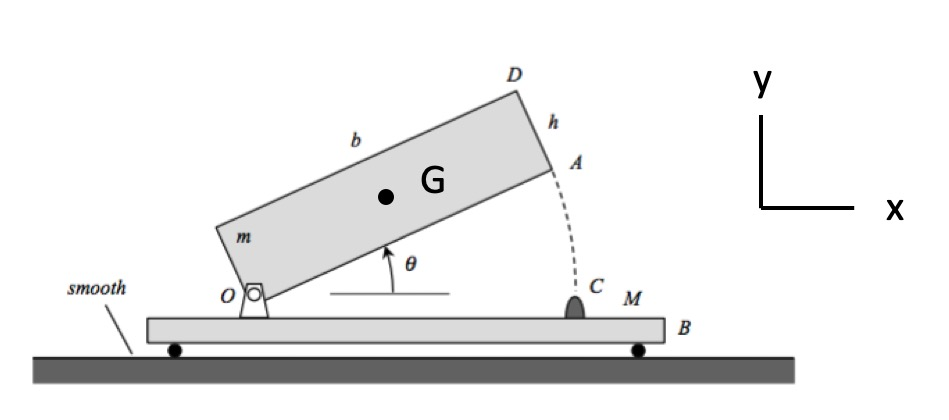# Linear momentum: rigid bodies

In a homework problem for this course, students are asked to consider the motion of a system made up of a plate (having a mass of m) which is pinned to a cart B (having a mass of M) at corner O. The system is released from rest with the center of mass of the plate displaced slightly to the right of the pin O. In describing the subsequent motion, it is expected that the students will use both:

• Conservation of energy between the times of impacts (since there is no non-conservative work being done between impacts).
• Conservation of linear momentum in the x-direction for ALL time (since no net horizontal force acts on the plate+cart system in that direction). Conservation of linear momentum in the x-direction gives the following result relating the velocities of the cart and the plate's COM, G:   vB = -(m/M) vGx. From this we know that the cart and G always move in opposite directions, and the speed of the cart is always proportional to the x-component of the velocity of G.General impact
An animation of this motion is shown below for a general value of the coefficient of restitution at the impact of A with C. From this we can easily verify that the cart and point G move in opposite directions:

• The cart initially moves to the left, and G moves to the right).
• After impact, the cart moves to the right and G moves to the left.Plastic impact
Suppose now we consider the case of a perfectly plastic impact of A with the bumper at C (COR = 0). What do we expect after impact? An immediate result is the the plate does not rebound from the bumper at C. Since the plate does not bounce back, vGx = 0. And from the above result for conservation of linear momentum, we see that vB = 0. In other words, the system comes to an abrupt stop. You can see this in the animation below for the COR = 0.

COR = 0